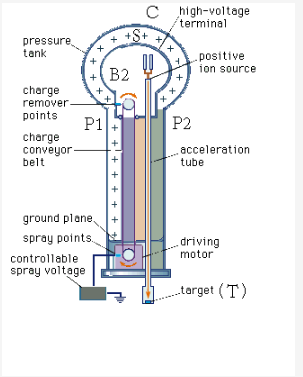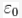# Explain construction and working of van-de-graff generator?

Explain construction and working of van-de-graff generator?

Van De Graff generator is used to generate high potential differences of near about million volts. Then the generated potential differences are used to speed up the particles like ions etc.

It is a device most commonly used in nuclear physics experiments.
In 1931 Van De Graff generator was designed.

Two main principles of Van De Graff Generator are given below:
� It is designed on the basis of the property that the charge given to a conductor which is hollow inside, is transferred to the outer surface and scatters uniformly over it.
� It is founded on the phenomenon of Corona Discharge.Construction of VanDeGraff generator: The diagram of Van DE Graff generator is shown below.
There is a large spherical conductor named S having a few meters of radius. It is placed on two pillars at a certain height from the ground. One is P1 and other is P2. A belt made up of insulating material is moving over two pulleys P1 and P2. The pulley P2 is present at the center of the spherical conductor S and the pulley P1 is present near the ground. A motor is used whose main function is to create a spin in the belt. Two sharp headed combs, B1 and B2 are used. Spray Comb and collecting comb are the names of B1 and B2 respectively. A discharge tube D is used in which the acceleration of ions is done. The point from where the ions originate is present at the head end of the discharge tube. But the other end of the tube is earthed.

The whole apparatus is placed in a steel compartment. Compartment is filled with Nitrogen or Methane. The pressure inside the chamber is maintained very high.

What is the working of a Van De Graff generator?
The initial start given to generator is by giving the first comb B1 a positive potential by a strong source of near about 104 Volt. Electric wind having a positive charge will be produced. Production of wind occurs due to the discharging of charge from the sharp edges. Belt moves continuously and will reach the sphere on moving. When the belt will reach the sphere an induced negative charge will be produced on the sharp edges of the comb B2. At once a induced positive charge will be produced on the other side of the comb B2. After this shifting of the charge, it will be transferred to sphere S. Similarly, due to the discharging action of the B2, wind will be produced but this will be negatively charged. The negatively charged wind helps to make the positively charged belt neutral. After this, the belt will be totally discharged. Again after rotation, the belt will come down. It will take the positive charge from the comb B1. Then again this charge will be taken by the comb B2.

The above process repeats again and again. Due to the repetition, the charge will start collecting on the sphere S.

Charge carrying capacity of the sphere will be shown with the formula.
= 4πR
In the above eqation, R is the radii of the spherical shell.
As we know that V= Q/C
Therefore V=Q/ 4 πR
From the equation it is clear that the potential and charge are directly proportional to each other. As we will increase the charge the V i.e. potential will also increase.

The air present around the sphere S will start ionizing when the potential of the spherical belt will cross the value of 3×106. 3×106 is the value of the air�s breakdown field. When the ionization of air starts then side by side leakage of charge will also take place. As we have discussed earlier that the generator is packed into a steel compartment filled with gas such as methane or nitrogen. So, leakage is minimized by this steel chamber.
Definition in brief: Let V be the potential difference present along the discharge tube and q be the charge present on the ion which is to be accelerated then the energy obtained by the ions will be given by: qV. This is the energy with which the ions strike the aim.

Things to Remember
� When capacitors are connected in parallel, and both the plates of capacitor are joined with the common battery. Then the potential difference passed by each each capacitor will be same.
� Charge on a capacitor may or may not be same. It depends upon the capacity of the capacitor.
� In a series combination there is only one route for the movement of charge.
� Due to higher capacity earth can take unlimited of charge.